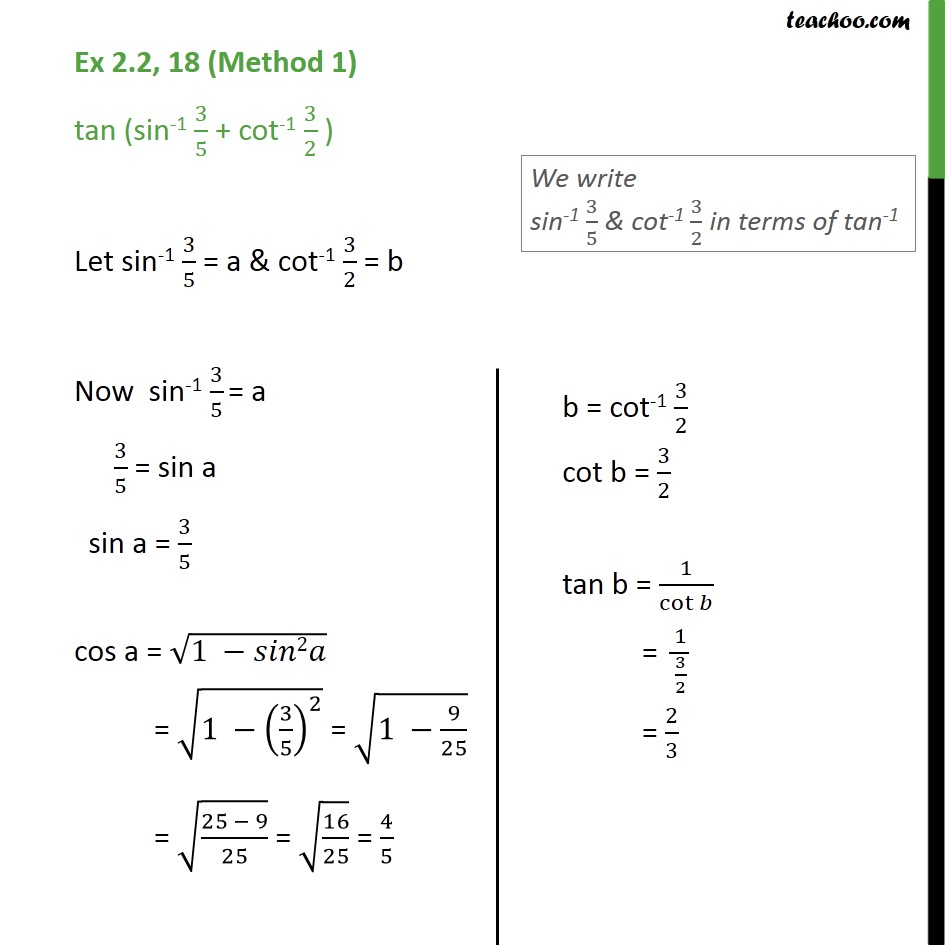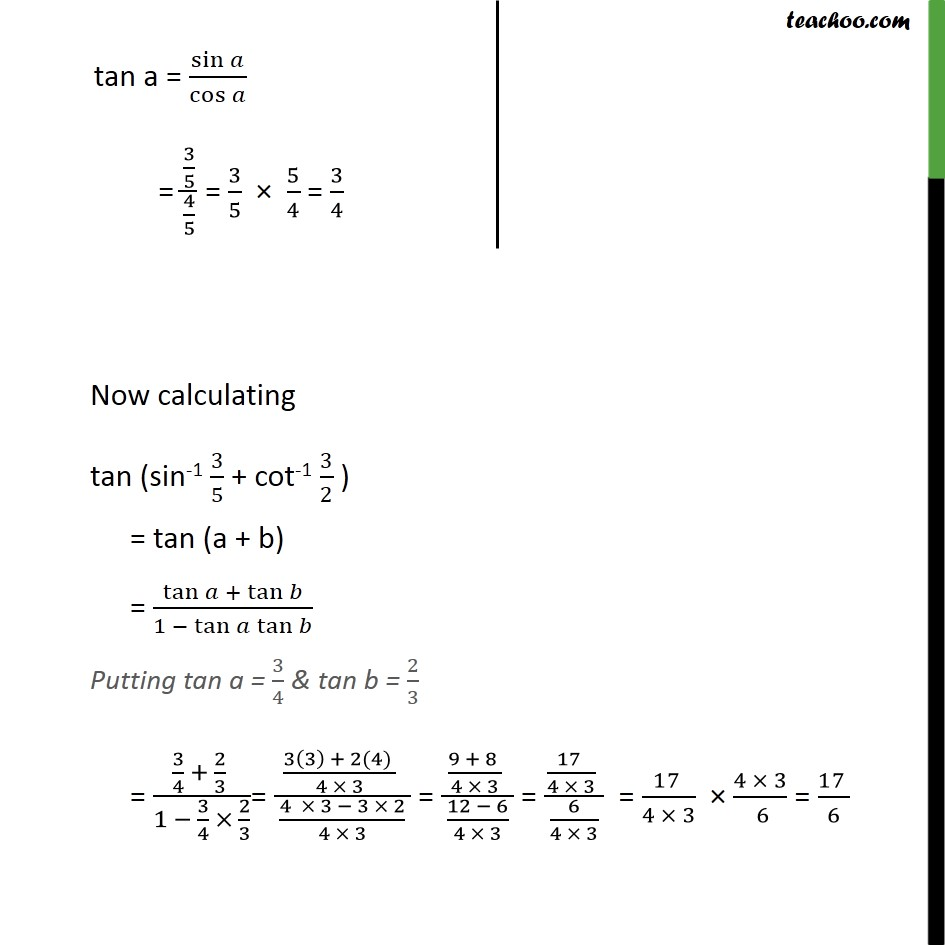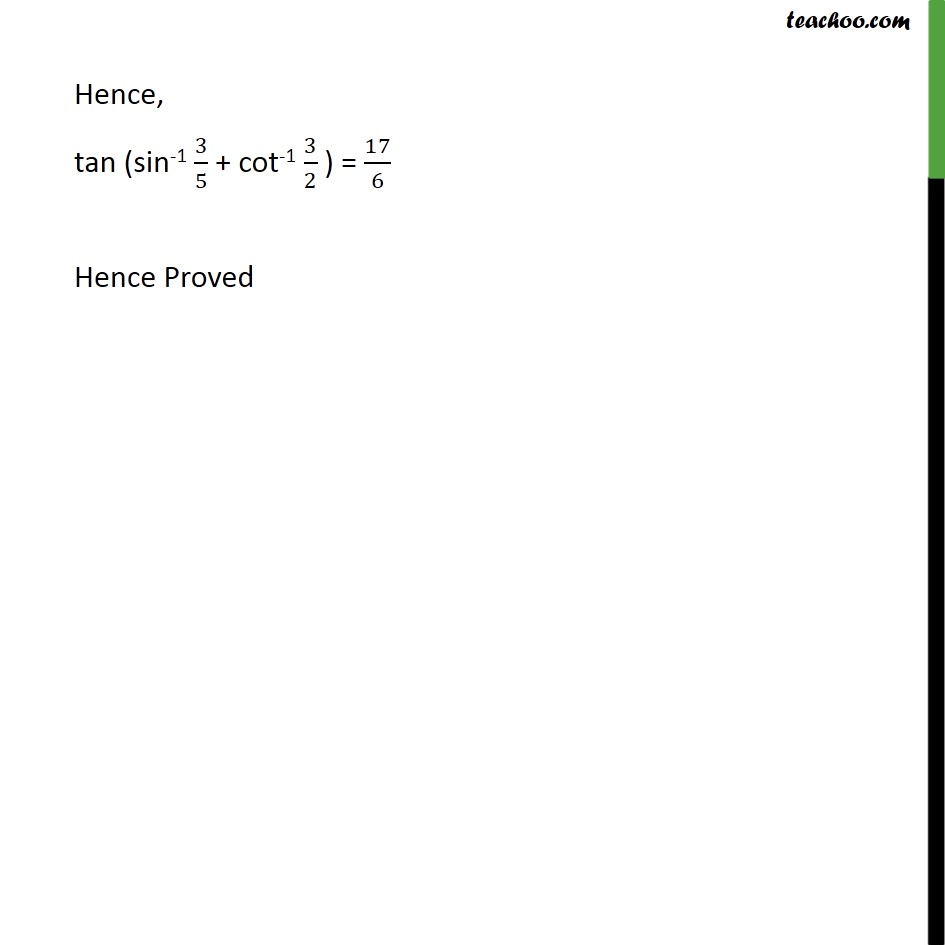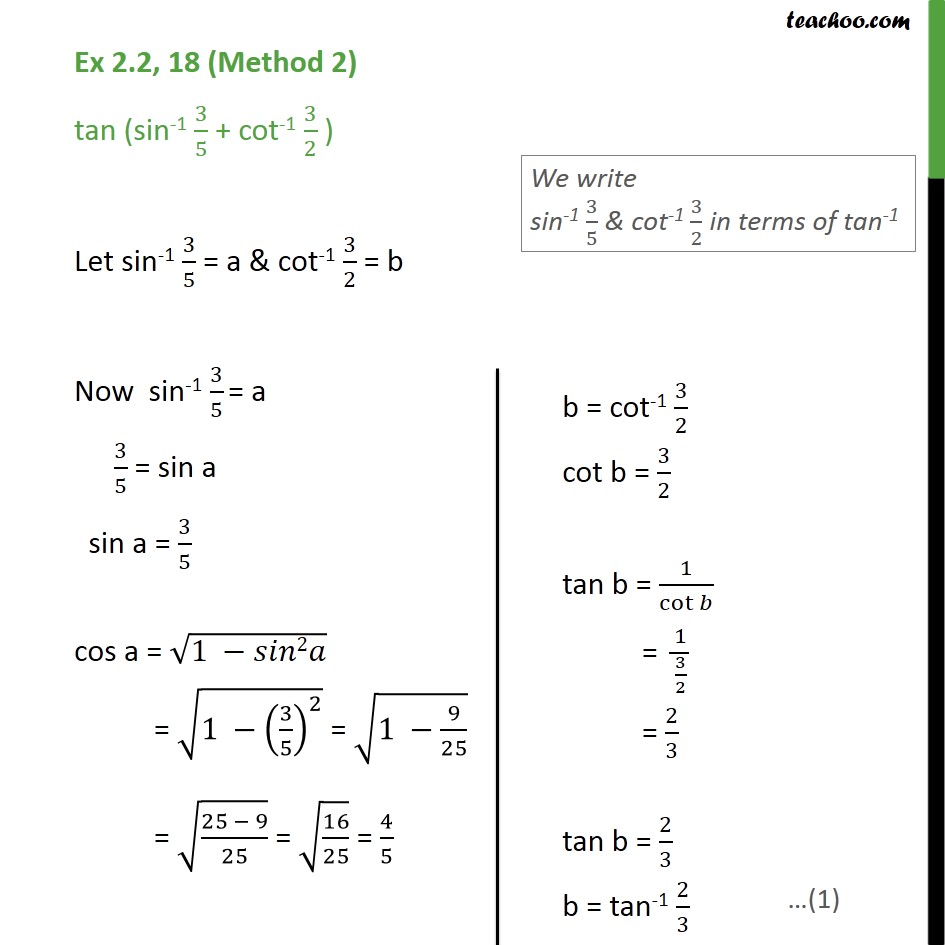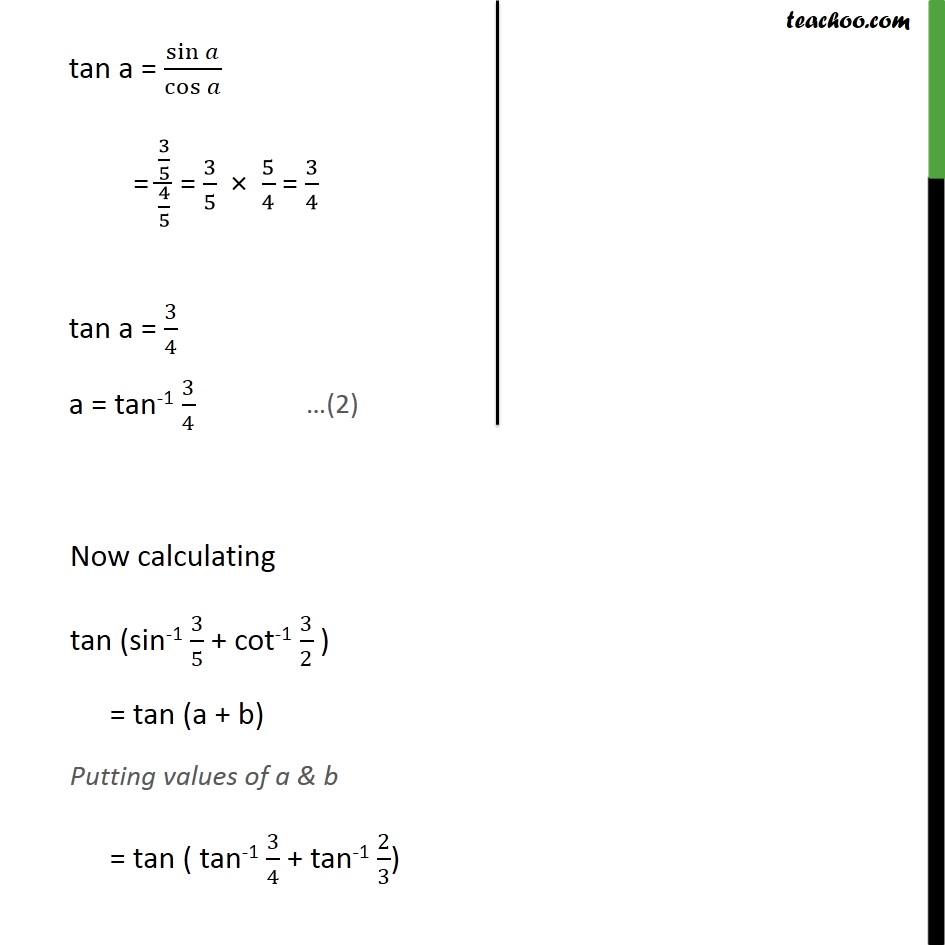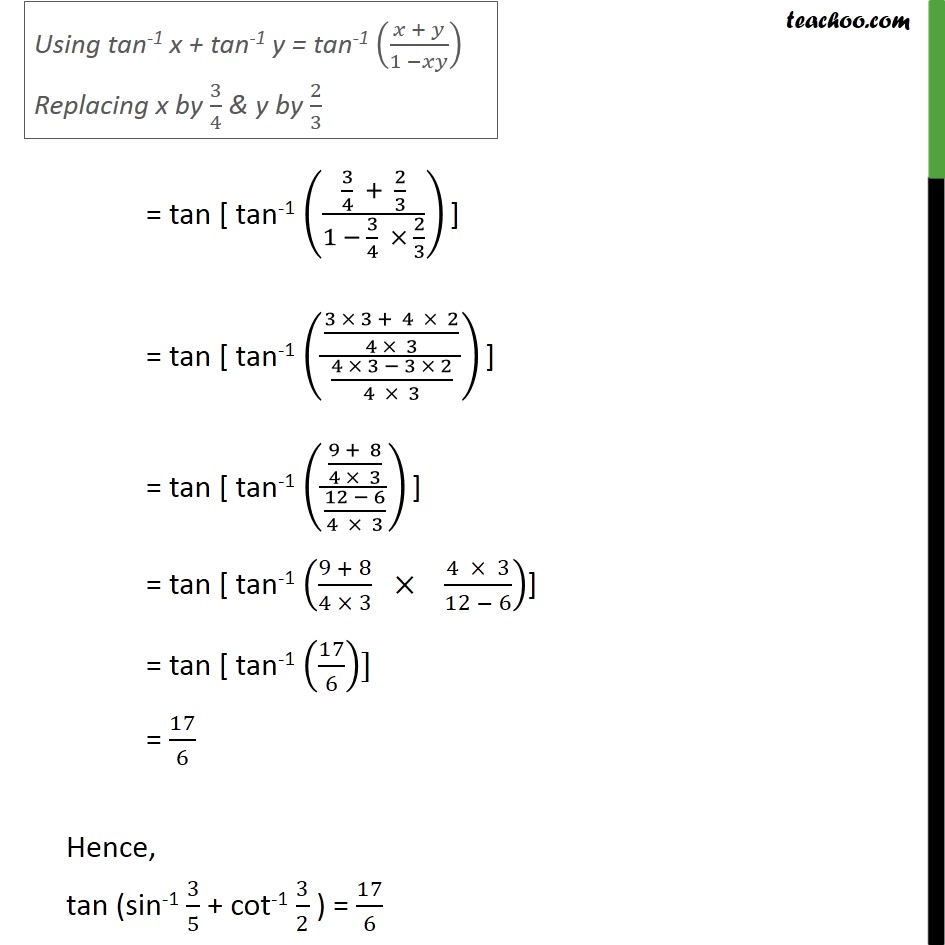1. Chapter 2 Class 12 Inverse Trigonometric Functions
2. Serial order wise
3. Ex 2.2

Transcript

Ex 2.2, 18 (Method 1) tan (sin-1 3/5 + cot-1 3/2 ) Let sin-1 3/5 = a & cot-1 3/2 = b Now sin-1 3/5 = a 3/5 = sin a sin a = 3/5 cos a = √(1 −𝑠𝑖𝑛2𝑎) = √(1 −(3/5)^2 ) = √(1 −9/25) = √((25 − 9)/25) = √(16/25) = 4/5 tan a = sin⁡𝑎/cos⁡𝑎 = (3/5)/(4/5) = 3/5 × 5/4 = 3/4 Hence, tan (sin-1 3/5 + cot-1 3/2 ) = 17/6 Hence Proved Ex 2.2, 18 (Method 2) tan (sin-1 3/5 + cot-1 3/2 ) Let sin-1 3/5 = a & cot-1 3/2 = b Now sin-1 3/5 = a 3/5 = sin a sin a = 3/5 cos a = √(1 −𝑠𝑖𝑛2𝑎) = √(1 −(3/5)^2 ) = √(1 −9/25) = √((25 − 9)/25) = √(16/25) = 4/5 tan a = sin⁡𝑎/cos⁡𝑎 = (3/5)/(4/5) = 3/5 × 5/4 = 3/4 tan a = 3/4 a = tan-1 3/4

Ex 2.2